1．矢量微分

[矢函数]  对于自变量t(标量)的每一个数值都有变动矢量a的确定量(长度与方向都确定的一个矢量)和它对应，则变(矢)量a称为变量t的矢函数，记作

af(t)

a=axiajazk

axfx(t)ayf(t)azfz(t)

若把变动矢量表成点M的矢径形式

rr(t)

r =xiyjzk

xx(t)yy(t)zz(t)

[矢函数的极限与连续性]  若对任意给定的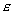>0 , 都存在数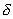>0，使得当|tt０|<|r(t)r|<= r0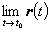存在，则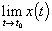i+j+k= r(t0),则称矢函数r(t)tt处连续.

[矢函数的导数与微分]  如果极限dadt

[矢函数求导公式]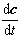0        (c为常矢量)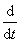(ka)k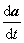(k为常数)(abc)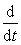(a)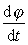a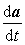(t的标函数)(ab)ba(顺序可以交换)(a×b)×ba×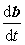(顺序不可以交换)(abc)=(bc)+(ac)+(ab)  (顺序不可以交换)

`a [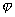(t)]=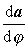`

(t的标函数，这是复合函数的求导公式)

[矢径形式的矢函数求导公式]

rr(t)x(t)iy(t)jz(t)k

1=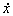i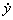j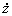k2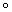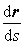= t

3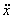i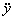j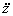k

[矢函数的泰勒公式]

r(tt)r(t)(t)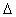t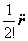(t)(t)＋・・・＋r(n)(t)(t)n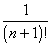rn(t)n+1

rnx(n+1)(t1)iy(n+1)(t2)jz(n+1)(t3)k    (t < t1 , t2 , t3 < tt)

r(n)(t)= x(n)(t)iy(n)(t)jz(n)(t)k

x(n)=, y(n)=, z(n)=[矢量函数的几个常用性质]

1定长矢量r(t)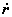(t)，反之也真.从而切线的单位矢量的导数与原矢量垂直.

2定向矢量r(t)//(t)，反之也真.

3一个变动矢量r(t)平行于一个定平面的充分必要条件是：混合积

(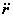)0